Processing ......FreeComputerBooks.com Links to Free Computer, Mathematics, Technical Books all over the World

Statistics with R
🌠 Top Free Programming Books - 100% Free or Open Source!
• Title Statistics with R
• Author(s) Vincent Zoonekynd, Jose Gama
• Publisher: Vincent Zoonekynd and Abo Akademi University; eBook (Creative Commons Licensed)
• Hardcover/Paperback: N/A
• eBook: HTML and PDF
• Language: English
• ISBN-10: N/A
• ISBN-13: N/ABook Description

This book provides an elementary-level introduction to R, targeting both non-statistician scientists in various fields and students of statistics.

The main mode of presentation is via code examples with liberal commenting of the code and the output, from the computational as well as the statistical viewpoint. Brief sections introduce the statistical methods before they are used. A supplementary R package can be downloaded and contains the data sets.

All examples are directly runnable and all graphics in the text are generated from the examples. The statistical methodology covered includes statistical standard distributions, one- and two-sample tests with continuous data, regression analysis, one-and two-way analysis of variance, regression analysis, analysis of tabular data, and sample size calculations. In addition, the last four chapters contain introductions to multiple linear regression analysis, linear models in general, logistic regression, and survival analysis.

• N/A

Reviews and Rating: Related Book Categories: Read and Download Links:Similar Books:
•Learning Statistics with R (Daniel Navarro)

This book takes you on a guided tour of software development with R, from basic types and data structures to advanced topics like closures, recursion, and anonymous functions. No statistical knowledge is required.

•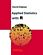Applied Statistics with R (David Dalpiaz)

This book provides an integrated treatment of statistical inference techniques in data science using the R Statistical Software. It provides a much-needed, easy-to-follow introduction to statistics and the R programming language.

•A Handbook of Statistical Analyses Using R (Brian S. Everitt, et al)

This book is the perfect guide for newcomers as well as seasoned users of R who want concrete, step-by-step guidance on how to use the software easily and effectively for nearly any statistical analysis.

•Introduction to Probability and Statistics Using R (G. Jay Kerns)

This is a textbook for an undergraduate course in probability and statistics, covers various basic and advanced statistical procedures, from correlation and graph creation to analysis of variance, regression, non-parametric tests, and more.

•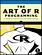The Art of R Programming: A Tour of Statistical Software Design

A guided tour of software development with R, from basic types and data structures to advanced topics like closures, recursion, and anonymous functions. No statistical knowledge is required, and your programming skills can range from hobbyist to pro.

•Efficient R Programming: Practical Guide to Smarter Programming

This book is about increasing the amount of work you can do with R in a given amount of time. It's about both computational and programmer efficiency. This book is for anyone who wants to make their use of R more reproducible, scalable, and faster.

•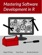Mastering Software Development in R (Roger D. Peng, et al.)

The book covers R software development for building data science tools. As the field of data science evolves, it has become clear that software development skills are essential for producing useful data science results and products.

•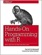Hands-On Programming with R: Functions and Simulations

This book not only teaches you how to program, but also shows you how to get more from R than just visualizing and modeling data. You’ll gain valuable programming skills and support your work as a data scientist at the same time.

•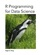R Programming for Data Science (Roger D. Peng)

This book is about the fundamentals of R programming. Get started with the basics of the language, learn how to manipulate datasets, how to write functions, and how to debug and optimize code. You will have a solid foundation on data science toolbox.

•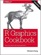R Graphics Cookbook: Practical Recipes for Visualizing Data

This cookbook provides more than 150 recipes to help scientists, engineers, programmers, and data analysts generate high-quality graphs quickly - without having to comb through all the details of R's graphing systems.

Book Categories
 :All CategoriesTop Free BooksRecent BooksMiscellaneous BooksComputer EngineeringComputer LanguagesComputer ScienceData Science/DatabasesJava and Java EE (J2EE)Linux and UnixMathematicsMicrosoft and .NETMobile ComputingNetworking and CommunicationsSoftware EngineeringSpecial TopicsWeb Programming
Other Categories